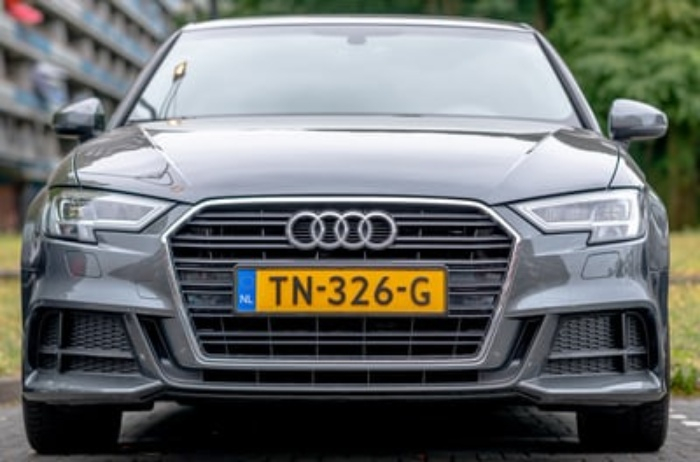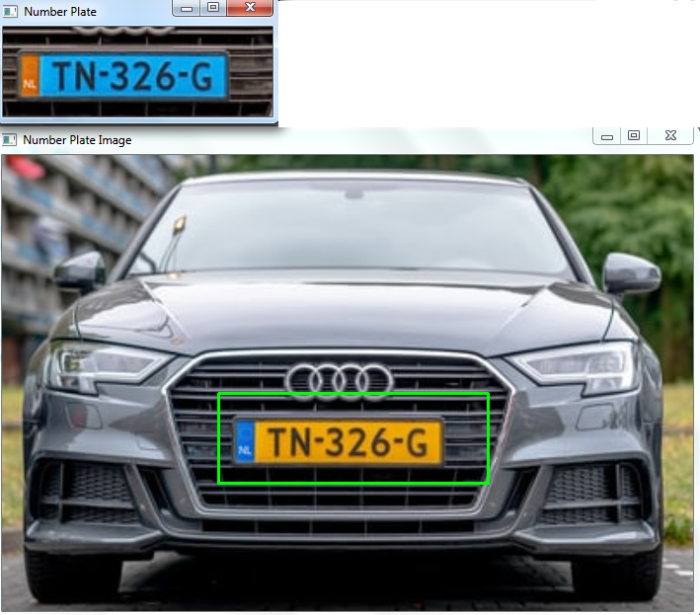# How to detect license plates using OpenCV Python?

We will use the Haar cascade classifier to detect the license number plate in the image. A haar cascade classifier is an effective object detection method. It is a machine learning based approach.

To train a number plate classifier, the algorithm initially needs a lot of positive images (images of number plates) and negative images (images without number plates). The classifier is trained from these positive and negative images. It is then used to detect objects (number plates) in other images. We can use already trained haar cascades for object detection.

## Steps

To detect license number plate in an image, we could follow the below steps-

• Import the required library. In all the following examples, the required Python library is OpenCV. Make sure you have already installed it.

• Read the input image using cv2.imread(). Specify the full image path. Convert the image to grayscale image.

• Initiate the Haarcascade classifier object plate_cascade = cv2.CascadeClassifier() for number plate detection. Pass the full path of the haar cascade xml files. You can use the haar cascade file haarcascade_russian_plate_number.xml to detect the number plate in the image.

• Detect the number plate in the input image using plate_cascade.detectMultiScale(). It returns the coordinates of the detected number plate in (x,y,w,h) format.

• Draw the bounding rectangles around the detected number plate in the original image using cv2.rectangle().

• Display the image with the drawn bounding rectangles around the license number plate.

Let's have a look at some examples for more clear understanding.

## Example

In this Python program, we detect license number plates in the input image using a haarcascade.

# import required libraries
import cv2
import numpy as np

# convert input image to grayscale
gray = cv2.cvtColor(img, cv2.COLOR_BGR2RGB)

print('Number of detected license plates:', len(plates))

# loop over all plates
for (x,y,w,h) in plates:

# draw bounding rectangle around the license number plate
cv2.rectangle(img, (x,y), (x+w, y+h), (0,255,0), 2)
gray_plates = gray[y:y+h, x:x+w]
color_plates = img[y:y+h, x:x+w]

# save number plate detected
cv2.imwrite('Numberplate.jpg', gray_plates)
cv2.imshow('Number Plate', gray_plates)
cv2.imshow('Number Plate Image', img)
cv2.waitKey(0)
cv2.destroyAllWindows()


We will use this image as the Input File for this program −## Output

On execution, it will produce the following output

Number of detected license plates: 1


And we get the following output window −